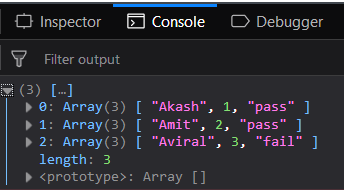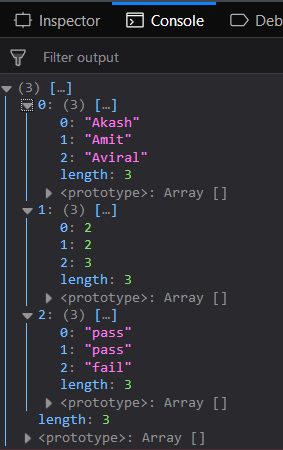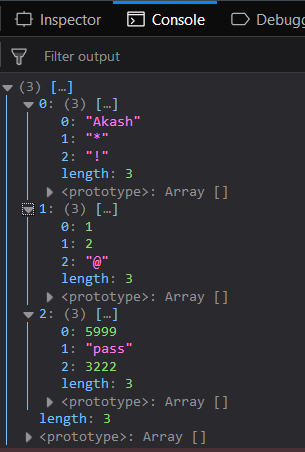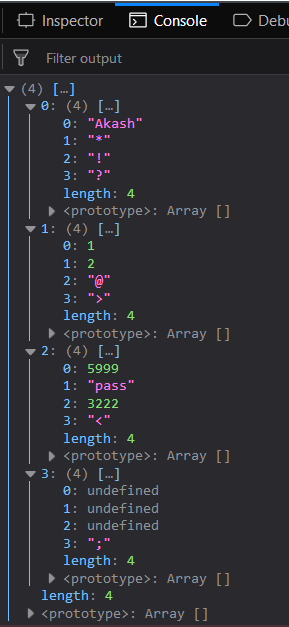Related Articles
Underscore.js | _.unzip() with Examples
• Last Updated : 22 Oct, 2020

The Underscore.js is a JavaScript library that provides a lot of useful functions like the map, filter, invokes, etc even without using any built-in objects.
The _.unzip() function is used to combine the same type of elements of different arrays into a single array. It matches one by one each passed array elements, to the next passed array elements. It is used when more than one homogeneous arrays (one type of elements like only numbers, works, special characters, etc) to connect all the array by matching each element to its corresponding element in the next array. It can have a number of arrays as its parameters. The result will contain the same number of arrays as the number of elements the largest array has. It is opposite to the _.zip() function.

Syntax:

`_.unzip( *arrays )`

Parameters: This function accepts single parameter array which is used to hold array elements.

Return value: It returns the resultant arrays.

Passing homogeneous arrays to _.unzip() function: The ._unzip() function accepts the element from the first array and makes the first elements of the resultant array. Then, it takes the elements from the second array and maps it as the second property of that first element only. This is done till all the arrays first elements are covered. Then it forms the second element of the resultant array. This goes on until all the elements are covered.

Example:

 ` ` `<``html``> ` `    ``<``head``> ` `        ``<``script` `src` `=  ` `"https://cdnjs.cloudflare.com/ajax/libs/underscore.js/1.9.1/underscore-min.js"` `> ` `        `` ` `    `` ` `    ``<``body``> ` `        ``<``script` `type``=``"text/javascript"``> ` `            ``console.log(_.unzip([['Akash', 'Amit', 'Aviral'],  ` `                                 ``[01, 02, 03],  ` `                                 ``['pass', 'pass', 'fail']]) ` `            ``); ` `        `` ` `    `` ` `                     `

Output:Passing a heterogeneous array to the _.unzip() function: Pass a heterogeneous array, i.e., which contains all kinds of elements in a single array. The _.unzip() function will work the same way. The procedure is the same but the output will be the difference. It will now form homogeneous arrays in the result.

Example:

 ` ` `<``html``> ` `    ``<``head``> ` `        ``<``script` `src` `=  ` `"https://cdnjs.cloudflare.com/ajax/libs/underscore.js/1.9.1/underscore-min.js"` `> ` `        `` ` `    `` ` `    ``<``body``> ` `        ``<``script` `type``=``"text/javascript"``> ` `            ``console.log(_.unzip([['Akash', 02, 'pass'],  ` `                                 ``['Amit', 02, 'pass'], ` `                                 ``['Aviral', 03, 'fail']]) ` `            ``); ` `        `` ` `    `` ` `                     `

Output:Mapping difference kinds of elements in _.unzip() function Pass different kind of elements to the _.unzip function. This time also the process will be the same but the result will not be a homogeneous array rather it will be a heterogeneous array as a string of words passed as the first element of the first array but a string of special characters is passed as the first element of the second array.

Example:

 ` ` `<``html``> ` `    ``<``head``> ` `        ``<``script` `src` `=  ` `"https://cdnjs.cloudflare.com/ajax/libs/underscore.js/1.9.1/underscore-min.js"` `> ` `        `` ` `    `` ` `    ``<``body``> ` `        ``<``script` `type``=``"text/javascript"``> ` `            ``console.log(_.unzip([['Akash', 01, 5999],  ` `                                 ``['*', 02, 'pass'],  ` `                                 ``['!', '@', 3222]]) ` `            ``); ` `        `` ` `    `` ` `                     `

Output:Passing unequal size of arrays to the _.unzip() function: One can pass unequal sized arrays but this will cause undefined values in the resultant arrays. This is because the number of elements who will find their matches in the rest of the arrays will be considered as a single array but if they do not found any match then they will have ‘undefined’ in place. But this will work smoothly and not give any error.

Example:

 ` ` `<``html``> ` `    ``<``head``> ` `        ``<``script` `src` `=  ` `"https://cdnjs.cloudflare.com/ajax/libs/underscore.js/1.9.1/underscore-min.js"` `> ` `        `` ` `    `` ` `    ``<``body``> ` `        ``<``script` `type``=``"text/javascript"``> ` `            ``console.log(_.unzip([['Akash', 01, 5999],  ` `                                 ``['*', 02, 'pass'],  ` `                                 ``['!', '@', 3222], ` `                                 ``['?', '>', '<', ';']]) ` `            ``); ` `        `` ` `    `` ` `                     `

Output:Note: These commands will not work in Google console or in Firefox as for these additional files need to be added which they didn’t have added. So, add the given links to your HTML file and then run them.

 `<``script` `type``=``"text/javascript"` `src` `=  ` `"https://cdnjs.cloudflare.com/ajax/libs/underscore.js/1.9.1/underscore-min.js"``>  ` `  `My Personal Notes arrow_drop_up
Recommended Articles
Page :